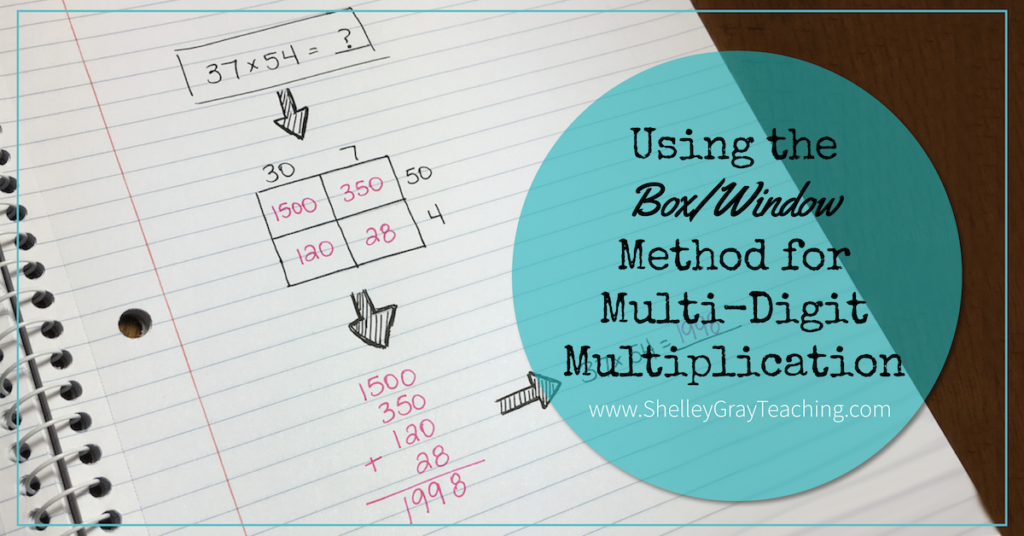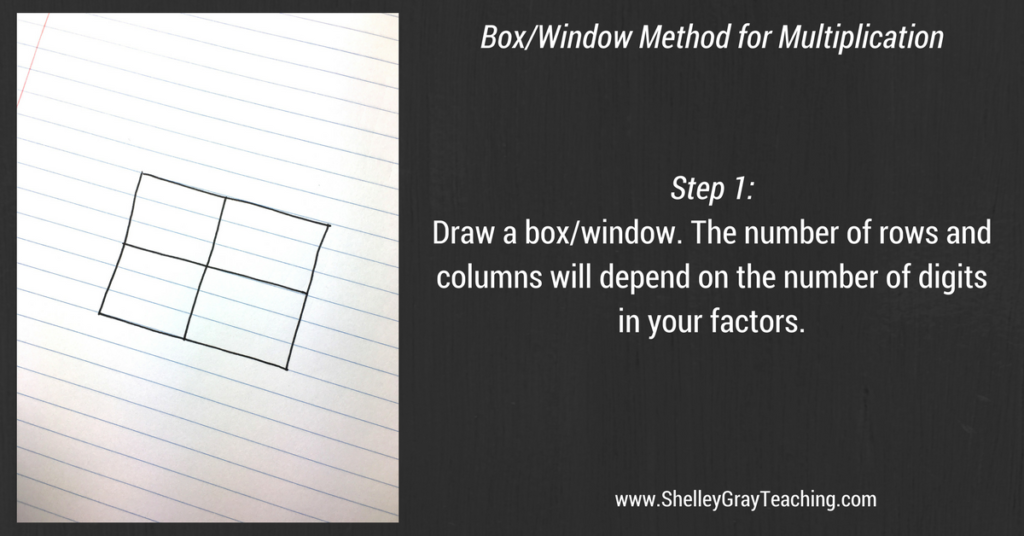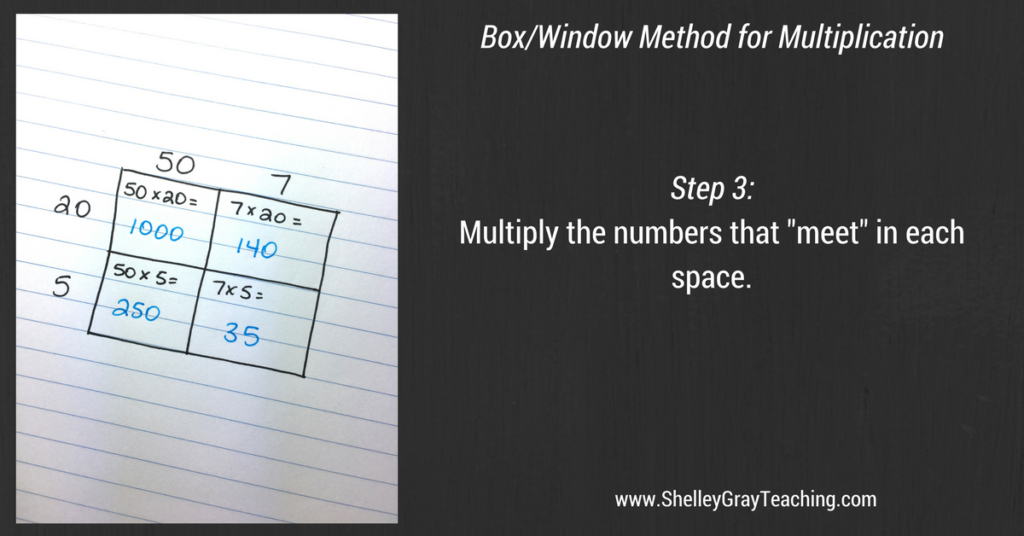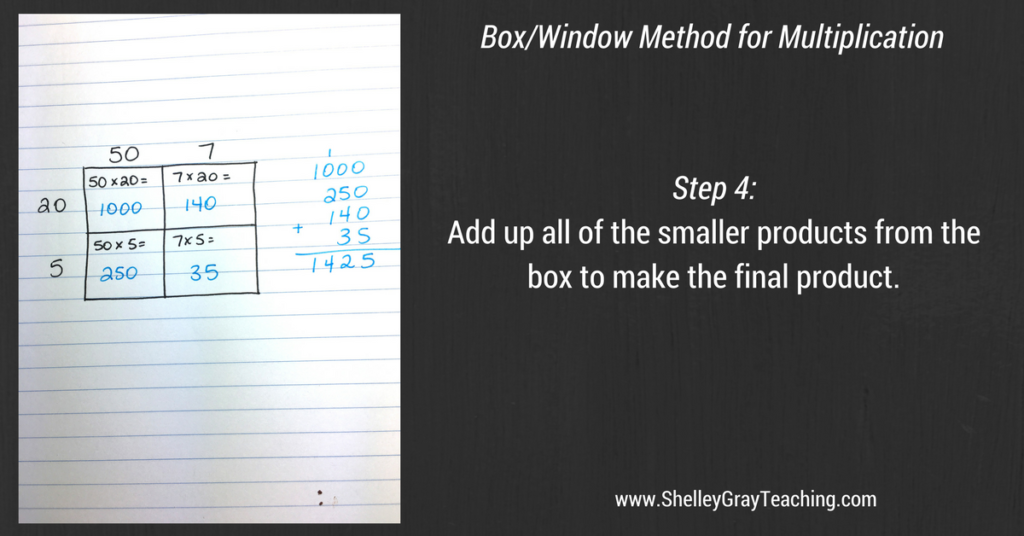# Using the Box/Window Method for Multi-Digit MultiplicationThe box/window method is an excellent strategy for multi-digit multiplication. This strategy places an emphasis on number sense understanding, as students use the expanded form of each factor. This helps students to understand what the digits in each factor REALLY mean.

This approach is also referred to as the “area model” by some teachers.

I’ve included detailed, step-by-step instructions for this method down below. However, if you would like to watch the steps in video format instead, simply press play on the video below. Otherwise, keep scrolling down to the step-by-step instructions!

I like the box/window method as an introduction to the partial products strategy. Although this basically is partial products, it is a more visual way to do the multiplication. Place value is emphasized because each factor is broken up into its expanded form, as shown below.When we use the box/window method, we first draw a box or window! The number of rows and columns will depend on the number of digits in the factor. The one below is drawn for a 2-digit by 2-digit equation; therefore there are two rows and two columns.Next, we arrange the expanded form of our factors along the top and one side of the box. For example, the box shown below is for the equation 57×25.Now it’s time to multiply! We multiply the numbers that meet in each space on the box. The picture below shows the numbers that are being multiplied in each box. Once your students are used to using this strategy, they will not need to write the entire equation in each box anymore, but can just write the products instead.Lastly we add all of the smaller products, to make our final product. In this example, the smaller products are added up to make 1425. This means that the product of 57 and 25 is 1425.When you use this strategy with larger numbers, you simply increase the size of the box to make room for more rows and columns. This is also an effective strategy for 2-digit by 1-digit multiplication, simply by making a rectangle divided into two separate sections, and finding the “area” of each section.

Looking for more help with multi-digit multiplication in your classroom? Check out my self-paced, student-centered Multi-Digit Multiplication Station HERE. It includes many different strategies for multi-digit multiplication so that each of your students can experience success in his own way.